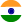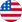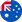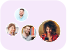24*7 support on WhatsAppChat NowProduct Edition:2nd Edition
Author: Tom M. Apostol
Book Name: Calculus, Vol. 1: One-Variable Calculus, with an Introduction to Linear Algebra
Subject Name: Maths

# Calculus, Vol. 1: One-Variable Calculus, with an Introduction to Linear Algebra 2nd Edition Solutions

0 out of 5.0
39 reviews37 Students
have requested for homework help from this book

An introduction to the Calculus, with an excellent balance between theory and technique. Integration is treated before differentiation--this is a departure from most modern texts, but it is historically correct, and it is the best way to establish the true connection between the integral and the derivative. Proofs of all the important theorems are given, generally preceded by geometric or intuitive discussion. This Second Edition introduces the mean-value theorems and their applications earlier in the text, incorporates a treatment of linear algebra, and contains many new and easier exercises. As in the first edition, an interesting historical introduction precedes each important new concept.

5
20
4
8
3
3
2
2
1
6

0

## Students who viewed this book also checked out